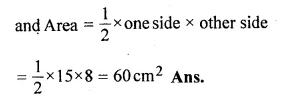Guru

# The length (in cm) of the hypotenuse of a right-angled triangle exceeds the length of one side by 2 cm and exceeds twice the length of another side by 1 cm. Find the length of each side. Also, find the perimeter and the area of the triangle.

• 0

This question has been taken from Book:- ML aggarwal, Avichal publication, class10th, quadratic equation in one variable, chapter 5, exercise 5.5
This is an important ques

The length (in cm) of the hypotenuse of a right-angled triangle exceeds the length of one side by 2 cm and exceeds twice the length of another side by 1 cm.

Find the length of each side.

Also, find the perimeter and the area of the triangle.
Question no45. , ML Aggarwal, chapter 5, exercise 5.5, quadratic equation in one variable, ICSE board,

Share